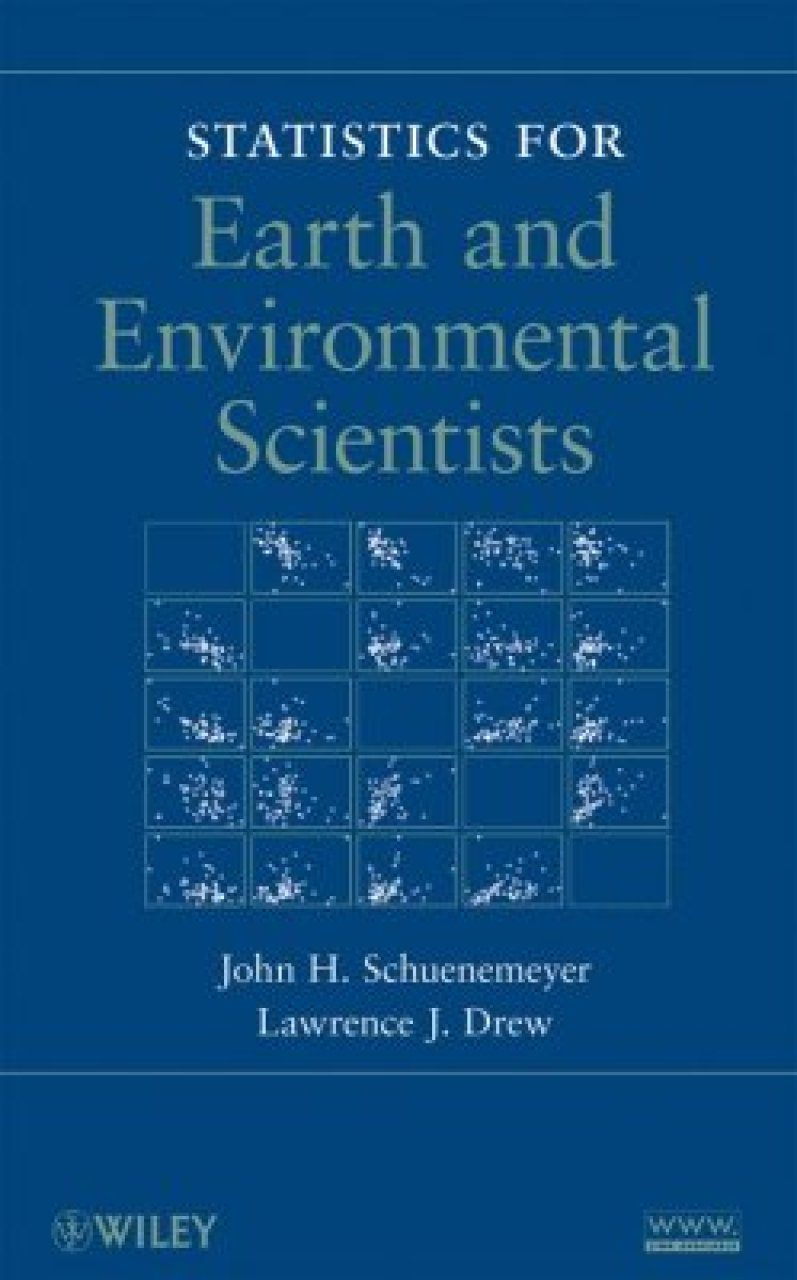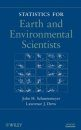## British Wildlife

8 issues per year 84 pages per issue Subscription only

British Wildlife is the leading natural history magazine in the UK, providing essential reading for both enthusiast and professional naturalists and wildlife conservationists. Published eight times a year, British Wildlife bridges the gap between popular writing and scientific literature through a combination of long-form articles, regular columns and reports, book reviews and letters.

Subscriptions from £40 per year

## Conservation Land Management

4 issues per year 44 pages per issue Subscription only

Conservation Land Management (CLM) is a quarterly magazine that is widely regarded as essential reading for all who are involved in land management for nature conservation, across the British Isles. CLM includes long-form articles, events listings, publication reviews, new product information and updates, reports of conferences and letters.

Subscriptions from £18 per year
• Huge product rangeOver 140,000 books & equipment products

• Rapid shippingUK & Worldwide

• Pay in £, € or U.S.\$By card, cheque, transfer, draft

• Exceptional customer serviceGet specialist help and advice

# Statistics for Earth and Environmental Scientists

By: John Schuenemeyer and Larry Drew
420 pages, figsClick to have a closer look
•ISBN: 9780470584699 Hardback Jan 2011 Usually dispatched within 4 days
£108.00
#189563

The goal of this book is to present skills and knowledge of important statistical concepts through data analytic tools and then apply them to real world problems in the environmental sciences. Since there is no single approach that works best in all research circumstances, the authors introduce models using the frequentist approach, but also discuss Bayesian, nonparametric, and computer intensive methods.

The book begins with an introduction to types of data, evaluation of data, modeling and estimation, random variation, sampling - all of which are explored in the context of case studies, which use real data from earth science applications. Subsequent chapters focus on general principles of modeling (namely formulation, estimation, and evaluation), interval estimation and hypothesis testing of means; regression; methods for analyzing time series data; spatial statistics; multivariate analysis; discrete distributions; and experimental design.

Most statistical models are introduced by concept and application, given as equations, and then accompanied by heuristic justification rather than a formal proof. In addition, graphs throughout the book illustrate concepts, identify unusual observations, and assist in model evaluation.

Each chapter concludes with exercises that are designed to elicit understanding of the processes of data analysis, model building, and statistical inference. Solutions to most problems are available on the book's related Web site, along with additional data sets and R-code for most graphs and analyses.

## Contents

Chapter 1. Role of statistics and data analysis. 1.1 Introduction. 1.2 Case studies. 1.3 Data. 1.4 Samples versus the population, some notation. 1.5 Vector and matrix notation. 1.6 Frequency distributions and histograms 1.7 The distribution as a model. 1.8 Sample moments. 1.9 Normal (Gaussian) distribution. 1.10 Exploratory data analysis. 1.11 Estimation. 1.12 Bias. 1.13 Causes of variance. 1.14 About data. 1.15 Reasons to conduct statistically based studies. 1.16 Data mining. 1.17 Modeling. 1.18 Transformations. 1.19 Statistical concepts. 1.20 Statistics paradigms. 1.21 Summary. 1.22 Exercises. Chapter 2. Modeling concepts. 2.1 Introduction. 2.2 Why construct a model? 2.3 What does a statistical model do? 2.4 Steps in modeling. 2.5 Is a model a unique solution to a problem? 2.6 Model assumptions. 2.7 Designed experiments. 2.8 Replication. 2.9 Summary. 2.10 Exercises. Chapter 3. Estimation and hypothesis testing on means and other statistics. 3.1 Introduction. 3.2 Independence of observations. 3.3 The Central Limit Theorem. 3.4 Sampling distributions. 3.4.1 t-distribution. 3.5 Confidence interval estimate on a mean. 3.6 Confidence interval on the difference between means. 3.7 Hypothesis testing on means. 3.8 Bayesian hypothesis testing. 3.9 Nonparametric hypothesis testing. 3.10 Bootstrap hypothesis testing on means. 3.11 Testing multiple means via analysis of variance. 3.12 Multiple comparisons of means. 3.13 Nonparametric ANOVA. 3.14 Paired data. 3.15 Kolmogorov-Smirnov goodness-of-fit test. 3.16 Comments on hypothesis testing. 3.17 Summary. 3.18 Exercises. Chapter 4. Regression. 4.1 Introduction. 4.2 Pittsburgh coal quality case study. 4.3 Correlation and covariance. 4.4 Simple linear regression. 4.5 Multiple regression. 4.6 Other regression procedures. 4.7 Nonlinear models. 4.8 Summary. 4.9 Exercises. Chapter 5. Time series. 5.1 #Introduction. 5.2 Time Domain. 5.3 Frequency Domain. 5.4 Wavelets. 5.5 Summary. 5.6 Exercises. Chapter 6. Spatial statistics. 6.1 Introduction. 6.2 Data. 6.3 Three-dimensional data visualization. 6.4 Spatial association. 6.5 The effect of trend. 6.6 Semivariogram models. 6.7 Kriging. 6.8 Space-time models. 6.9 Summary. 6.10 Exercises. Chapter 7. Multivariate analysis. 7.1 Introduction. 7.2 Multivariate graphics. 7.3 Principal component analysis. 7.4 Factor analysis. 7.5 Cluster analysis. 7.6 Multidimensional scaling. 7.7 Discriminant analysis. 7.8 Tree based modeling. 7.9 Summary. 7.10 Exercises. Chapter 8. Discrete data analysis and point processes. 8.1 Introduction. 8.2 Discrete process and distributions. 8.3 Point processes. 8.4 Lattice data and models. 8.5 Proportions. 8.6 Contingency tables. 8.7 Generalized linear models. 8.8 Summary. 8.9 Exercises. Chapter 9 Design of experiments. 9.1 Introduction. 9.2 Sampling designs. 9.3 Design of experiments. 9.4 Comments on field studies and design. 9.5 Missing data. 9.6 Summary. 9.7 Exercises. Chapter 10 Directional data. 10.1 Introduction. 10.2 Circular data. 10.3 Spherical data. 10.4 Summary. 10.5 Exercises.

## Biography

John H. Schuenemeyer, PhD, is President of Southwest Statistical Consulting, LLC and Professor Emeritus of Statistics, Geography, and Geology at the University of Delaware. A Fellow of the American Statistical Association, Dr. Schuenemeyer has more than thirty years of academic and consulting experience and was the recipient of the 2004 John Cedric Griffiths Teaching Award, awarded by the International Association for Mathematical Geosciences. Lawrence J. Drew, PhD, is Research Scientist at the U.S. Geological Survey. Dr. Drew has published more than 200 scientific papers on the role of quantitative methods in petroleum and mineral resource assessment, and he is currently is working on an analysis of environmental data. Dr. Drew is the winner of the 2005 Krumbein Medal, awarded by the International Association for Mathematical Geosciences.

By: John Schuenemeyer and Larry Drew
420 pages, figs
Media reviews

Proper collection and analysis of data using statistical techniques contributes significantly toward the solution of these problems. Statistics for Earth and Environmental Scientists presents important statistical concepts through data analytic tools and shows readers how to apply them to real-world problems. (Breitbart.com: Business Wire, 2 March 2011)